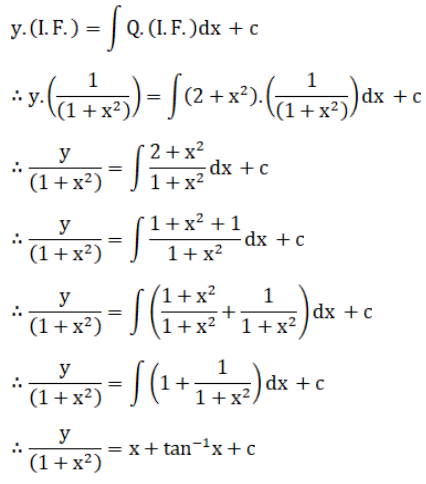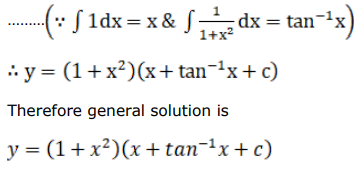# Find the general solution for each of the following differential equations.

Question:

Find the general solution for each of the following differential equations.

$\left(x^{2}+1\right) \frac{d y}{d x}-2 x y=\left(x^{2}+1\right)\left(x^{2}+2\right)$

Solution: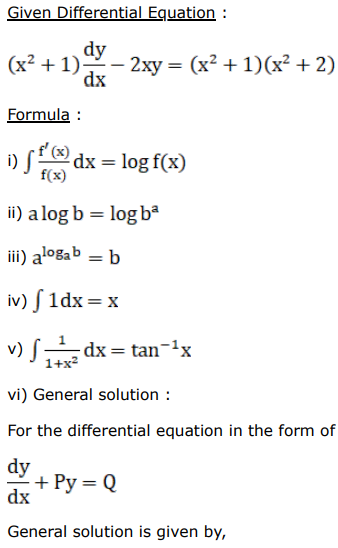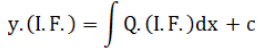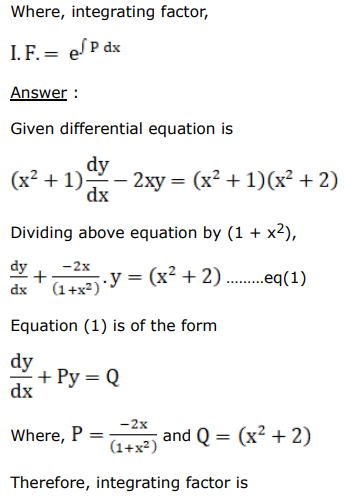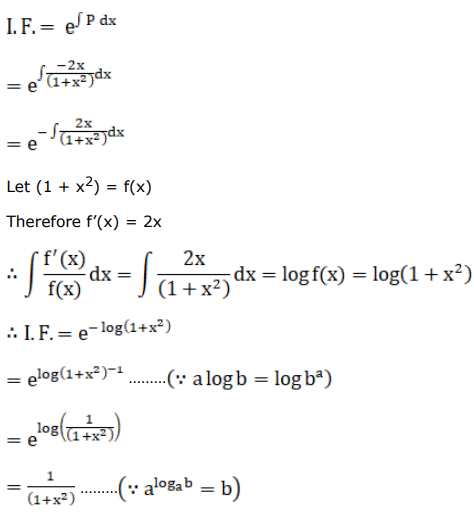General solution is